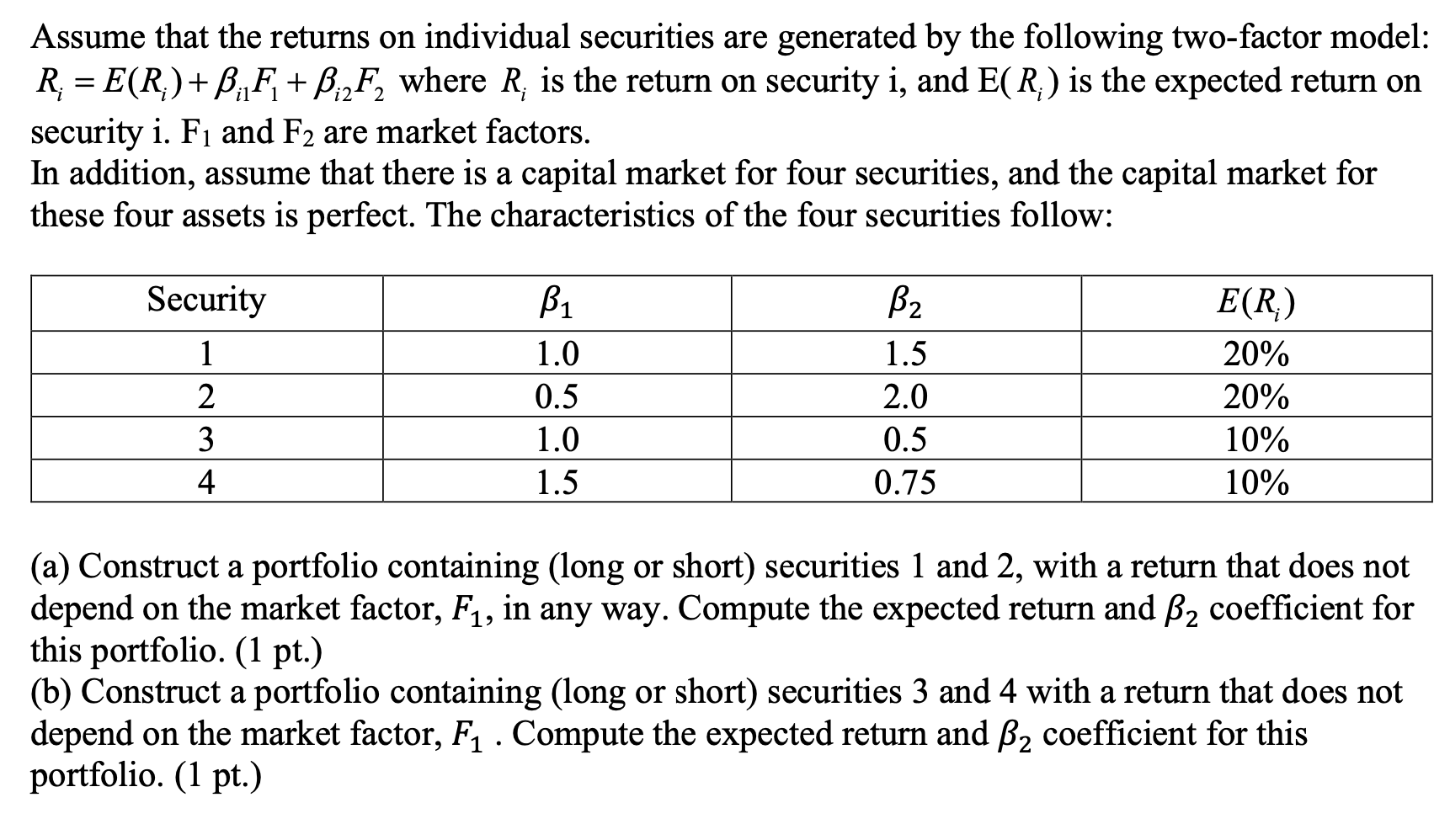# Question Solved1 AnswerAssume that the returns on individual securities are generated by the following two-factor model: R2 = E(R)+B:Fi + PizF, where R, is the return on security i, and E(R) is the expected return on security i. Fi and F2 are market factors. In addition, assume that there is a capital market for four securities, and the capital market for these four assets is perfect. The characteristics of the four securities follow: Security 1 2 3 4 B1 1.0 0.5 1.0 1.5 B2 1.5 2.0 0.5 0.75 E(R) 20% 20% 10% 10% (a) Construct a portfolio containing (long or short) securities 1 and 2, with a return that does not depend on the market factor, F1, in any way. Compute the expected return and B2 coefficient for this portfolio. (1 pt.) (b) Construct a portfolio containing (long or short) securities 3 and 4 with a return that does not depend on the market factor, F1. Compute the expected return and B2 coefficient for this portfolio. (1 pt.)Transcribed Image Text: Assume that the returns on individual securities are generated by the following two-factor model: R2 = E(R)+B:Fi + PizF, where R, is the return on security i, and E(R) is the expected return on security i. Fi and F2 are market factors. In addition, assume that there is a capital market for four securities, and the capital market for these four assets is perfect. The characteristics of the four securities follow: Security 1 2 3 4 B1 1.0 0.5 1.0 1.5 B2 1.5 2.0 0.5 0.75 E(R) 20% 20% 10% 10% (a) Construct a portfolio containing (long or short) securities 1 and 2, with a return that does not depend on the market factor, F1, in any way. Compute the expected return and B2 coefficient for this portfolio. (1 pt.) (b) Construct a portfolio containing (long or short) securities 3 and 4 with a return that does not depend on the market factor, F1. Compute the expected return and B2 coefficient for this portfolio. (1 pt.)
More
Transcribed Image Text: Assume that the returns on individual securities are generated by the following two-factor model: R2 = E(R)+B:Fi + PizF, where R, is the return on security i, and E(R) is the expected return on security i. Fi and F2 are market factors. In addition, assume that there is a capital market for four securities, and the capital market for these four assets is perfect. The characteristics of the four securities follow: Security 1 2 3 4 B1 1.0 0.5 1.0 1.5 B2 1.5 2.0 0.5 0.75 E(R) 20% 20% 10% 10% (a) Construct a portfolio containing (long or short) securities 1 and 2, with a return that does not depend on the market factor, F1, in any way. Compute the expected return and B2 coefficient for this portfolio. (1 pt.) (b) Construct a portfolio containing (long or short) securities 3 and 4 with a return that does not depend on the market factor, F1. Compute the expected return and B2 coefficient for this portfolio. (1 pt.)